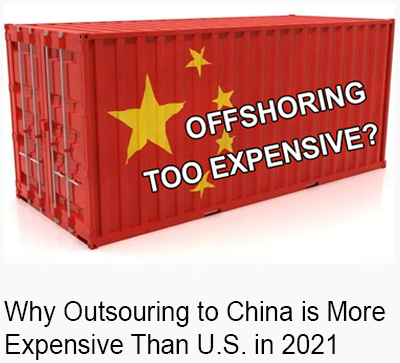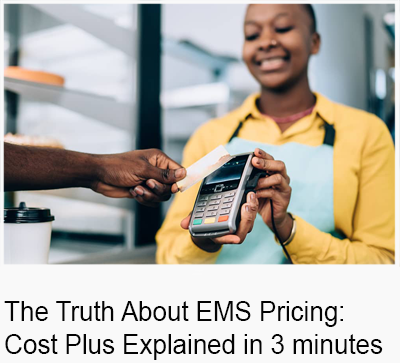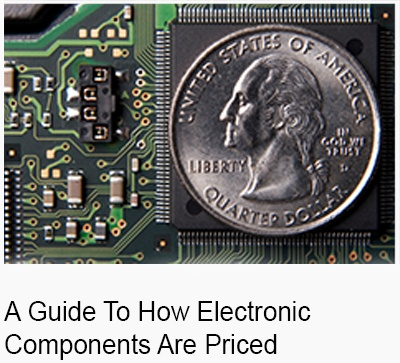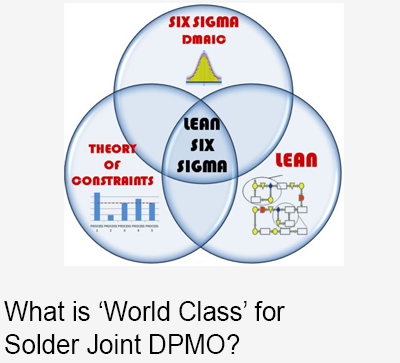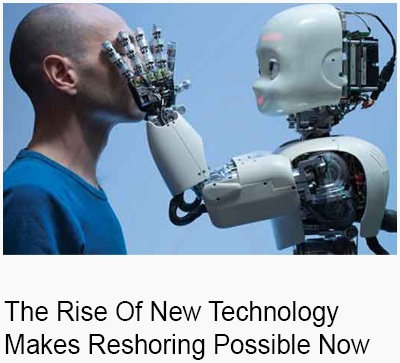New product development builds are more expensive because of the small lot sizes and quick turnarounds.  But how much more? Here's a formula:

Sum the cost of materials multiplied by a factor for attrition, plus \$1 per placement, then divide the sum by .65.

Material + (Material x Attrition Factor) + (# of Placements)
___________________________________

.65

Figuring out the material value is straightforward, except for the fabs (raw, unpopulated PCBs). Fab prices vary wildly based on how fast you want them and how small a quantity you order. Often you will see quotes of \$1000 for 1pc, \$500 for 2pc, \$250 for 4pc, etc., which is essentially a lot charge. If you need the boards in 1–3 days, expect to pay a massive premium, 5 days a premium of approximately 20–50 percent, a small premium for a 10-day turn, and no premium for 15 days or more. Fab suppliers can estimate cost if they know your layer count, board dimensions, material type, finish, and copper weight.

The attrition factor accounts for the loss of material when loading the equipment and for component purchase minimums (which are very common). It is expressed as a percentage of the material cost. This is another difficult number, but here is the rule of thumb we use:

10–15, and 10–12%

15–20, less than 8–10%

20–30, 5%

30 and up, 2%To estimate the labor and overhead charge, figure \$1 per placement. This charge can also vary considerably; \$1 is conservative, 50 cents is optimistic. You can quickly calculate the number of placements by simply adding the Qty Per column on the BOM; in other words, the total number of placements is equal to the sum of quantity per’s.

The profit margin you should expect to pay is about 35 percent. To calculate selling price, divide costs by 1 – Profit Margin, so to calculate 35 percent margin you divide cost by .65 (1 - .35).

If the costs seem high, realize that most CMs do not make money running NPI; that’s why you so often have trouble getting attention paid to your NPI projects. A CM will always make more money running a volume repeat job than by running a first-time, low-volume NPI job, no matter how much they charge for NPI.

## SUBSCRIBE NOW